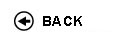Politecnico di Torino
Academic Year 2017/18
02KXUJM
Mathematical analysis II
1st degree and Bachelor-level of the Bologna process in Mechanical Engineering - Torino
 Teacher Status SSD Les Ex Lab Tut Years teaching Como GiacomoA2 ING-INF/04 50 30 0 0 2
 SSD CFU Activities Area context MAT/05 6 A - Di base Matematica, informatica e statistica
 Subject fundamentals This course first completes the theory of functions of one variable which was developed in the Mathematical Analysis course, introducing the student to basic concepts of numerical series, power series, Fourier series, and Laplace transform. The course then introduces basic topics in the mathematical analysis of functions of several variables including differential calculus in several variables, the theory of multiple integration, line, and surface integration. Expected learning outcomes Knowledge: - Definition, properties, and convergence criteria for numerical series. - Definition, properties, and convergence criteria for sequences and series of functions, power series, and Fourier series. - Definition and main properties of Laplace transform. - Properties and analysis of functions of several variables. - Definition and properties of double, triple, line, and surface integrals. - Conservative vector field; Green, Gauss, and Stokes theorems. Ability to: - classify the asymptotic behaviour (e.g., converge or the lack thereof) of numerical series, sequences of functions, and series of functions using the criteria introduced in class; - infer the set and type of convergence of sequences and series of functions; - compute the power series approximation of a function and the Fourier series approximation of a periodic function; - compute the Laplace transform of simple functions and compare their properties; - analyze a function of several variables; find and classify its critical points; - compute double, triple, line, and surface integrals; - reproduce, discuss, and explain theoretical results presented in class and apply them to solve simple problems. Prerequisites / Assumed knowledge The topics covered in the Mathematical Analysis I and Linear Algebra and Geometry courses. In particular, notions and properties of limits, numerical sequences, differential and integral calculus for functions of one variable, differential equations, linear algebra, geometry of curves. Contents - Laplace transform. - Numerical series: definition and convergence criteria. Power series and Taylor series. Fourier series. - Review on vectors and elementary notions of topology of R^n. Functions of several variables, vector fields. Limits and continuity. Partial and directional derivatives, Jacobian matrix. Differentiability, gradient, and tangent plane. Second derivatives, Hessian matrix. Taylor polynomial. Critical points, unconstrained maxima and minima. - Double and triple integrals, center of mass. Length of a curve and area of the region under the graph of a function. Line and surface integrals (for graphs of functions only). Circulation and flux of a vector field. - Conservative vector fields. Green, Gauss, and Stokes theorems. Delivery modes Theoretical lectures and practice classes. Theoretical lectures are devoted to the presentation of the topics, with definitions, properties, introductory examples, as well as a number of selected proofs which are believed to facilitate the learning process. The practice classes are devoted to the acquisition of the abilities through guided solution of problems. Texts, readings, handouts and other learning resources The following textbook covers the topics of the course and will be used as a reference: - C. Canuto, A. Tabacco, "Mathematical Analysis II", Springer` Other material will be suggested in class and made avalaible thorugh the Portale della Didattica. Assessment and grading criteria The examination consists of a written test and, possibly, an oral test. The written test consists of a number of multiple choice questions and one or more problems with open-ended answer. Both parts are aimed at checking the acquired knowledge and abilities. Questions cover both theoretical aspects and the solution of simple problems, including the evaluation of integrals and series. The problem section is more complex and allows for a more precise evaluation of the abilities acquired by the student. The written test’s duration is two hours and it is closed-book: the use of any notes, books, exercise sheets, or pocket calculators is not allowed. The grade of the written test ranges from 0/30 to 30/30 and is the aggregate of the points assigned to the single answers to the multiple choice questions and the problems with open-ended answer. The maximum number of points that can be assigned to the answer of each question and problem are explicitly specified in the exam text. The oral test takes place only if required either by the teacher or by the student, in the latter case only if the student’s grade in the written test is larger than or equal to 18/30. The oral test aims at evaluating more in depth the knowledge and abilities acquired by the student and possibly clarifying issues raised by the written text: potential questions in the oral test may include the topics and problems covered in written test, however they are not limited to them and may span the whole course contents. In case the oral test takes place, the final grade ranges from 0/30 to 30L/30 and depends both on the written test’s grade and the student’s performance in the oral test. Programma definitivo per l'A.A.2017/18© Politecnico di Torino
Corso Duca degli Abruzzi, 24 - 10129 Torino, ITALY